# Class 10th Chemical Reaction and Equation

Class 10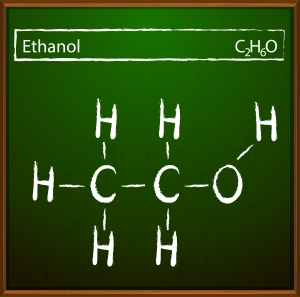Class 10th Chemical Reaction and Equation. refers to the transformation of one chemical substance into another chemical substance. Common examples of chemical reactions include the rusting of iron, the setting of milk into curd, digestion of food, and respiration. In this process, a new substance is formed that has entirely different properties compared to the original substance. Hence, a chemical change takes place during a chemical reaction. However, it is important to note that only a rearrangement of atoms occurs in such a reaction.

The substances that participate in a chemical reaction are called reactants. Conversely, the new substances produced as a result of a chemical reaction are called products. For instance, the burning of magnesium in the air to form magnesium oxide is an example of a chemical reaction. The chemical equation for this reaction is 2Mg(s) + O2(g) △→ 2MgO(s). Before the burning process, the magnesium ribbon is cleaned by rubbing with sandpaper to remove the protective layer of basic magnesium carbonate from its surface.

In conclusion, chemical reactions play an essential role in our daily lives. They occur naturally and artificially and are responsible for producing everything from energy to food. Understanding chemical reactions and their mechanisms is crucial for the development of new materials and technologies that can improve our lives.

### Chemical Reactions:

(i) When zinc reacts with dilute sulphuric acid, it evolves hydrogen gas. The chemical equation for this reaction is Zn(s) + H2SO4(aq) → ZnSO4(aq) + H2(g) ↑.

(ii) The reaction between citric acid and purple coloured potassium permanganate solution causes a change in colour from purple to colourless. Similarly, the reaction between sulphur dioxide gas and acidified potassium dichromate solution results in a change in colour from orange to green.

(iii) During the combustion of candle wax, the substance undergoes a change in state from solid to liquid and gas. This is because wax is a solid, and the water produced during the combustion is a liquid at room temperature, whereas carbon dioxide is a gas. Some chemical reactions exhibit multiple characteristics.

(iv) The chemical reaction between quicklime and water to form slaked lime is characterized by a rise in temperature. Similarly, the reaction between zinc granules and dilute sulphuric acid also results in a rise in temperature.

(v) When sulphuric acid reacts with barium chloride solution, it forms a white precipitate of barium sulphate. The chemical equation for this reaction is BaCl2(aq) + H2SO4(aq) → BaSO4(s) (ppt) + 2HCl(aq).

Chemical Reactions and Equations: Balanced and unbalanced chemical equations and balancing of chemical equations.

### What is a chemical reaction?- Class 10th Chemical Reaction and Equation

Chemical Reaction: The transformation of chemical substance into another chemical substance is known as Chemical Reaction. For example: Rusting of iron, the setting of milk into curd, digestion of food, respiration, etc.

In a chemical reaction, a new substance is formed which is completely different in properties from the original substance, so in a chemical reaction, a chemical change takes place.
Only a rearrangement of atoms takes place in a chemical reaction.

• The substances which take part in a chemical reaction are called reactants.
• The new substances produced as a result of a chemical reaction are called products.

Example: The burning of magnesium in the air to form magnesium oxide is an example of a chemical reaction.
2Mg(s) + O2(g)  2MgO(s)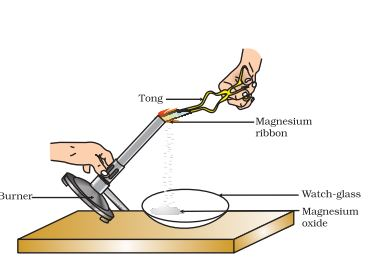Before burning in air, the magnesium ribbon is cleaned by rubbing with sandpaper.
This is done to remove the protective layer of basic magnesium carbonate from the surface of the magnesium ribbon.

Reactant: Substances which take part in a chemical reaction are called reactants.
Example: Mg and O2.

Product: New substance formed after a chemical reaction is called a product.
Example: MgO.

Characteristics of Chemical Reactions :
(i) Evolution of gas: The chemical reaction between zinc and dilute sulphuric acid is characterised by the evolution of hydrogen gas.
Zn(s) + H2SO4(aq) → ZnSO4(aq) + H2(g) ↑

(ii) Change in Colour: The chemical reaction between citric acid and purple coloured potassium permanganate solution is characterised by a change in colour from purple to colourless.
The chemical reaction between sulphur dioxide gas and acidified potassium dichromate solution is characterized by a change in colour from orange to green.

(iii) Change in state of substance: The combustion reaction of candle wax is characterised by a change in state from solid to liquid and gas (because the wax is a solid, water formed by the combustion of wax is a liquid at room temperature whereas, carbon dioxide produced by the combustion of wax is a gas). There are some chemical reactions which can show more than one characteristics.

(iv) Change in temperature: The chemical reaction between quick lime water to form slaked lime is characterized by a change in temperature (which is a rise in temperature).
The chemical reaction between zinc granules and dilute sulphuric acid is also characterised by a change in temperature (which is a rise in temperature).

(v) Formation of precipitate: The chemical reaction between sulphuric acid and barium chloride solution is characterised by the formation of a white precipitate of barium sulphate.
BaCl2(aq) + H2SO4(aq) → BaSO4(s) (ppt) + 2HCl(aq)

### What is a chemical Equation Class 10?- Class 10th Chemical Reaction and Equation

Chemical Equation is the representation of a chemical reaction using symbols and formulae of the substances involved.

For example, in the equation A + B → C + D, A and B are the reactants, and C and D are the products. The arrow indicates the direction of the chemical reaction, and any necessary conditions are typically written above it.

When hydrogen reacts with oxygen to form water, the chemical equation is written as: Hydrogen + Oxygen → Water or H2 + O2 → H2O. Symbols are used to represent the substances in this equation, whereas the first example uses words.

### Balanced chemical equations:

A chemical equation is a concise and informative way to represent a chemical reaction. There are two types of chemical equations: balanced and unbalanced.

(a) Write the unbalanced chemical equation with the correct chemical formulae for all the reactants and products.

(b) Count the number of atoms of each element present in reactants and products.

(c) Add coefficients before the chemical formulae of reactants and products to balance the number of atoms of each element.

(d) Check if the balanced chemical equation obeys the Law of Conservation of Mass.

### Unbalance equation:

For the given chemical equation: Fe + H2O → Fe3O4 + H2

(a) The unbalanced chemical equation is already given with the correct chemical formulae.

(b) Counting the number of atoms of each element, we get: Reactants: 1 Fe atom, 2 H atoms, 1 O atom Products: 3 Fe atoms, 2 H atoms, 4 O atoms

(c) Adding coefficients before the chemical formulae to balance the number of atoms of each element, we get: Fe + 4H2O → Fe3O4 + 4H2

(d) Checking if the balanced chemical equation obeys the Law of Conservation of Mass: Reactants: 1 Fe atom, 8 H atoms, 4 O atoms Products: 3 Fe atoms, 8 H atoms, 4 O atoms

The number of atoms of each element is equal on both sides, so the chemical equation is balanced and obeys the Law of Conservation of Mass.

Write the number of atoms of elements present in reactants and in products in a table as shown here.

 Name of atom No. of atoms in the reactant No. of atoms in the product Iron 1 3 Hydrogen 2 2 Oxygen 1 4
1. Identify the atom that is maximum in number on either side of the equation.
2. Balance that atom by multiplying the appropriate molecules or compounds on either side of the equation.
3. Check the equation to ensure that the number of atoms of each element is equal on both sides.
4. If the equation is not balanced, repeat steps 2 and 3 until the equation is balanced.

Example: Fe + H2O → Fe3O4 + H2

1. Oxygen is the atom that is maximum in number on the RHS.
2. Multiply H2O on the LHS by 4 to get 4 oxygen atoms on both sides. Fe + 4H2O → Fe3O4 + H2
3. There are 8 hydrogen atoms on the LHS and only 2 on the RHS. Multiply H2 by 4 on the RHS to get 8 hydrogen atoms on both sides. Fe + 4H2O → Fe3O4 + 4H2
4. There is 1 Fe atom on the LHS and 3 Fe atoms on the RHS. Multiply Fe on the LHS by 3 to get 3 Fe atoms on both sides. 3Fe + 4H2O → Fe3O4 + 4H2
5. The equation is now balanced with 3 Fe, 4 H2O, 1 Fe3O4, and 4 H2 on both sides.
 Name of atom No. of atoms in the reactant No. of atoms in the product Iron 3 3 Hydrogen 8 8 Oxygen 4 4

After balancing, the above equation can be written as follows:
3Fe + 4H2O → Fe3O4 + 4H2.

To make a chemical equation more informative, one can write the symbols of physical states of substances involved in the reaction. For instance, gaseous state is represented by the symbol (g), liquid state is represented by (l), solid state by (s), and aqueous solution by (aq).

In addition to the physical state symbols, writing the condition under which the reaction takes place can also enhance the informativeness of the equation. Generally, the condition is written above and/or below the arrow of the chemical equation.

Therefore, by incorporating physical state symbols and reaction conditions, a chemical equation can be made more informative.

### What are the types of a chemical reaction Class 10?

Types of Chemical Reactions: Class 10th Chemical Reaction and Equation let you understand different types of reactions such as Combination Reaction, Decomposition Reaction, Displacement Reaction, Double Displacement Reaction, Neutralization Reactions, Exothermic – Endothermic Reactions and Oxidation-Reduction Reactions.

### (i) Combination Reaction:

Reactions in which two or more reactants combine to form one product are called Combination Reactions.
A general combination reaction can be represented by the chemical equation given here:
A + B → AB
Examples:
When magnesium is burnt in the air (oxygen), magnesium oxide is formed. In this reaction, magnesium is combined with oxygen.
Mg(s) + O2(g) → 2MgO(s)

Magnesium + Oxygen → Magnesium Oxide

When carbon is burnt in oxygen (air), carbon dioxide is formed. In this reaction, carbon is combined with oxygen.
C (s) + O2(g) → CO2(g)
Carbon + Oxygen → Carbon dioxide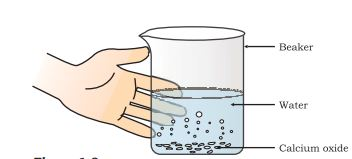### (ii) Decomposition Reaction:

Reactions in which a compound breaks down into two or more simpler substances are called Decomposition Reactions. A general decomposition reaction can be represented by the chemical equation given here: AB → A + B Examples: When calcium carbonate is heated, it decomposes to form calcium oxide and carbon dioxide. CaCO3(s) → CaO(s) + CO2(g) Calcium Carbonate → Calcium Oxide + Carbon Dioxide

When silver chloride is exposed to sunlight, it decomposes to form silver and chlorine gas. 2AgCl(s) → 2Ag(s) + Cl2(g) Silver Chloride → Silver + Chlorine

Chemical reactions that involve the breakdown of one compound into two or more compounds or elements are called Decomposition Reactions. The opposite of a combination reaction, a decomposition reaction can be represented as AB → A + B. Some examples of decomposition reactions include:

Heating calcium carbonate results in the production of calcium oxide and carbon dioxide. CaCO3(s) heat−→− CaO(s) + CO2(g) Calcium carbonate → Calcium oxide + Carbon dioxide

Heating ferric hydroxide causes it to decompose into ferric oxide and water. 2Fe(OH)3(s) △→ Fe2O3(s) + 3H2O(l)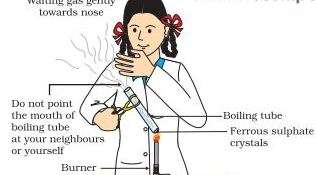Thermal Decomposition:

The decomposition of a substance on heating is known as Thermal Decomposition.
Example: 2Pb(NO3)2(s) heat−→− 2PbO(s) + 4NO2(g) + O2(g)

Electrolytic Decomposition: Reactions in which compounds decompose into simpler compounds because of passing of electricity, are known as Electrolytic Decomposition. This is also known as Electrolysis.
Example: When electricity is passed in water, it decomposes into hydrogen and oxygen.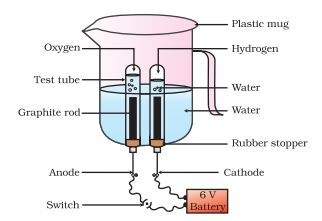Photolysis or Photo Decomposition Reaction: Reactions in which a compound decomposes because of sunlight are known as Photolysis or Photo Decomposition Reaction.
Example: When silver chloride is put in sunlight, it decomposes into silver metal and chlorine gas.
2AgCl(s) (white) Sunlight−→−−−−− 2Ag(s) (grey) + Cl2(g)

Photographic paper has a coat of silver chloride, which turns into grey when exposed to sunlight. It happens because silver chloride is colourless while silver is a grey metal.

### (iii) Displacement Reaction:

Displacement Reactions are chemical reactions where a more reactive element displaces a less reactive element from a compound. They are also known as Substitution Reactions or Single Displacement/Replacement Reactions.

• You can represent a general displacement reaction using a chemical equation in the following way: A + BC → AC + B.
• Displacement reactions occur only when ‘A’ is more reactive than B. If ‘B’ is more reactive than ‘A’, then ‘A’ will not displace ‘C’ from ‘BC,’ and the reaction will not occur.
• Examples of displacement reactions include:
• Zinc reacting with hydrochloric acid to produce hydrogen gas and zinc chloride: Zn(s) + 2HCl(aq) → ZnCl2(aq) + H2(g)
• Zinc reacting with copper sulphate to form zinc sulphate and copper metal: Zn(s) + CuSO4(aq) → ZnSO4(aq) + Cu(s)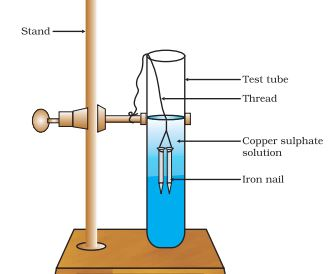### (iv) Double Displacement Reaction:

Double displacement reactions involve the exchange of ions between two reactants resulting in the formation of new compounds. The chemical equation for a double displacement reaction can be represented as AB + CD → AC + BD.

For instance, when a solution of barium chloride reacts with a solution of sodium sulphate, a white precipitate of barium sulphate is formed along with sodium chloride. The balanced chemical equation for this reaction is BaCl2(aq) + Na2SO4(aq) → BaSO4(s) (Precipitate) + 2NaCl(aq).

Similarly, when sodium hydroxide, which is a base, reacts with hydrochloric acid, it forms sodium chloride and water. The chemical equation for this reaction is NaOH(aq) + HCl(aq) → NaCl(aq) + H2O(l).

It is worth noting that double displacement reactions that involve the formation of a precipitate are also known as precipitation reactions. Neutralization reactions are also examples of double displacement reactions.

### Precipitation Reaction:

When two aqueous solutions of ionic compounds are mixed together, a precipitation reaction can occur. This reaction results in the formation of an insoluble solid compound, known as a precipitate.

Examples:

• Mixing a solution of silver nitrate (AgNO3) with a solution of sodium chloride (NaCl) results in the formation of a white precipitate of silver chloride (AgCl). AgNO3(aq) + NaCl(aq) → AgCl(s)↓ + NaNO3(aq)
• Mixing a solution of lead nitrate (Pb(NO3)2) with a solution of potassium iodide (KI) results in the formation of a yellow precipitate of lead iodide (PbI2). Pb(NO3)2(aq) + 2KI(aq) → PbI2(s)↓ + 2KNO3(aq)

Neutralization Reaction is a type of chemical reaction that occurs when an acid reacts with a base to form a salt and water. The exchange of ions takes place during this reaction. Some examples of neutralization reactions are:

When hydrochloric acid reacts with sodium hydroxide, sodium chloride (salt) and water are formed. HCl(aq) + NaOH(aq) → NaCl(aq) + H2O(l)

When sulphuric acid reacts with calcium hydroxide, calcium sulphate (salt) and water are formed. H2SO4(aq) + Ca(OH)2(aq) → CaSO4(aq) + 2H2O(l)

In both examples, an acid (HCl or H2SO4) reacts with a base (NaOH or Ca(OH)2) to produce a salt and water.

### (v) Oxidation and Reduction Reactions:

When a compound or element undergoes addition of oxygen or non-metallic element or removal of hydrogen or metallic element, it is known as Oxidation. The elements or compounds that experience this change are said to be oxidized.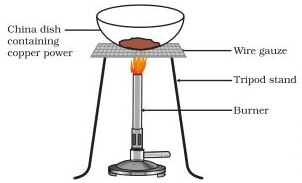On the other hand, when a compound or element undergoes addition of hydrogen or metallic element or removal of oxygen or non-metallic element, it is called Reduction. The compound or element which undergoes this change is said to be Reduced.

It is important to note that Oxidation and Reduction reactions occur simultaneously and are collectively known as Redox reactions.

Examples: When magnesium reacts with oxygen, it is oxidized to form magnesium oxide. 2Mg(s) + O2(g) → 2MgO(s) Magnesium + Oxygen → Magnesium Oxide

When copper (II) oxide reacts with hydrogen gas, it is reduced to form copper metal and water. CuO(s) + H2(g) → Cu(s) + H2O(l) Copper oxide + Hydrogen gas → Copper metal + Water

• The substance which gives oxygen for oxidation is called an Oxidizing agent.
• The substance which removes hydrogen is also called an Oxidizing agent.

### Reducing agent:

• The substance which gives hydrogen for reduction is called a Reducing agent.
• The substance which removes oxygen is also called a Reducing agent.

Redox reactions are reactions in which both oxidation and reduction take place simultaneously. For example, when hydrogen reacts with copper oxide, copper metal and water are formed. The chemical equation for this reaction is CuO + H2 → Cu + H2O.

In this reaction, copper oxide is reduced to copper, as oxygen is removed from the compound. The removal of oxygen is known as reduction. On the other hand, hydrogen is oxidized to water, as oxygen is added to the compound. The addition of oxygen is known as oxidation.

The substance that undergoes oxidation is the reducing agent, while the substance that undergoes reduction is the oxidizing agent.

### (vi) Exothermic and Endothermic Reactions:

Exothermic Reaction:An exothermic reaction is a reaction that releases energy in the form of heat or light. One example of an exothermic reaction is combustion, such as burning wood. Another example is the reaction between hydrochloric acid and sodium hydroxide: HCl(aq) + NaOH(aq) → NaCl(aq) + H2O(l) + heat

Respiration is actually an endothermic reaction because it absorbs energy from the surroundings rather than releasing it. During respiration, glucose and oxygen are consumed to produce carbon dioxide, water, and ATP energy for the cell. The equation for respiration is:

C6H12O6 + 6O2 → 6CO2 + 6H2O + ATP (energy)

Endothermic Reactions:Endothermic reactions absorb heat energy. One example of an endothermic reaction is the decomposition of calcium carbonate.

CaCO3(s) + heat energy -> CaO(s) + CO2(g)

In this reaction, calcium carbonate breaks down into calcium oxide and carbon dioxide. The reaction requires heat energy to be supplied for it to proceed, making it an endothermic reaction.

### Effects of Oxidation Reactions in Everyday life- Class 10th Chemical Reaction and Equation

Corrosion:Corrosion occurs when metals react with oxygen, water, acids, gases, etc. in the atmosphere, leading to their slow conversion into undesirable compounds. Rusting of iron is an example of corrosion, where iron reacts with oxygen and moisture to form a red substance called rust.

2Fe + O2 + H2O → Fe2O3.H2O (Rust)

The rusting of iron is a redox reaction.
Corrosion (rusting) weakens the iron and steel objects and structures such as railings, car bodies, bridges and ships etc. and cuts short their life.
Methods to Prevent Rusting

• By painting.
• By greasing and oiling.
• By galvanisation.

Corrosion of Copper: When copper objects are exposed to air, the surface of these objects reacts with moisture and carbon dioxide to form a green coating of basic copper carbonate, CuCO3.Cu(OH)2, causing the objects to lose their lustre and shine. This process is called corrosion.

Example: The Statue of Liberty in New York is made of copper and over time, it has acquired a green coating due to corrosion.

Equation: 2Cu + O2 + CO2 + H2O → CuCO3.Cu(OH)2

Rancidity: The taste and odour of food materials containing fat and oil changes when they are left exposed to air for a long time. This is called Rancidity. It is caused due to the oxidation of fat and oil present in food materials.

Methods to prevent rancidity:

• Vacuum packing.
• Replacing air by nitrogen.
• Refrigeration of foodstuff.

1. Chemical Reaction: During chemical reactions, the chemical composition of substances changes or new substances are formed.

2. Chemical Equation: Chemical reactions can be written in chemical equation form which should always be balanced.

3. Types of Chemical Reactions:

Combination reaction: A single product is formed from two or more reactants.
2Mg + O2 → 2MgO

Decomposition reaction: A single reactant breaks down to yield two or more products.

• Thermal decomposition: 2Pb(NO2)2 → 2PbO + 4NO2 + O2
• Electrolysis: 2H20 → 2H2 + O2
• Photochemical reaction: 2AgBr → 2Ag + Br2

Displacement reaction: One element is displaced by another element.
Zn + CuSO4 → ZnSO4 + Cu

Double displacement reaction: Exchange of ions between reactants.
AgNO3 + NaCl → AgCl + NaNO3

Redox reaction: Both oxidation and reduction take place simultaneously.
CuO + H2 → Cu + H2O

Exothermic reaction: A chemical reaction in which heat energy is evolved.
C + O2 → CO2 (g) + heat

Endothermic reaction: A chemical reaction in which heat energy is absorbed.
ZnCO3 + Heat → ZnO + CO2

Redox reaction: Chemical reaction in which both oxidation and reduction take place simultaneously.

4. Oxidation: Reaction that involves the gain of oxygen or loss of hydrogen.

5. Reduction: Reaction that shows the loss of oxygen or gain of hydrogen.
ZnO + C → Zn + CO
ZnO is reduced to Zn—reduction. C is oxidized to CO—Oxidation.

### Effects of Oxidation Reactions in Our Daily Life - Class 10th Chemical Reaction and Equation

• Corrosion: It is an undesirable change that occurs in metals when they are attacked by moisture, air, acids and bases.
Example, Corrosion (rusting) of Iron: Fe2O3. nH2O (Hydrated iron oxide)
• Rancidity: Undesirable change that takes place in oil containing food items due to the oxidation of fatty acids.
Preventive methods of rancidity: Adding antioxidants to the food materials, storing food in the airtight container, flushing out air with nitrogen gas and refrigeration.

### Conclusion

In conclusion, the topic of Class 10th Chemical Reaction and Equation is an essential aspect of the science curriculum. Through the study of chemical reactions and equations, students gain a deeper understanding of the world around them and how substances interact with one another. By grasping the fundamental principles of chemistry, students can apply their knowledge to everyday life, and even pursue careers in fields such as medicine, engineering, and research. Therefore, it is crucial that educators continue to prioritize the teaching of chemical reactions and equations in Class 10th and beyond, to equip students with the necessary knowledge and skills to succeed in their academic and professional endeavors.